# 逼着面试官问了我 ArrayList 和 LinkedList 的区别，他对我彻底服了### 01、ArrayList 是如何实现的？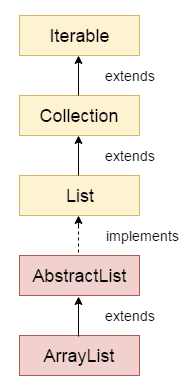ArrayList 实现了 List 接口，继承了 AbstractList 抽象类，底层是基于数组实现的，并且实现了动态扩容。

public class ArrayList<E> extends AbstractList<E>        implements List<E>, RandomAccess, Cloneable, java.io.Serializable{    private static final int DEFAULT_CAPACITY = 10;    transient Object[] elementData;    private int size;}

ArrayList 还实现了 RandomAccess 接口，这是一个标记接口：

public interface RandomAccess {}

public E get(int index) {    Objects.checkIndex(index, size);    return elementData(index);}E elementData(int index) {    return (E) elementData[index];}

ArrayList 还实现了 Cloneable 接口，这表明 ArrayList 是支持拷贝的。ArrayList 内部的确也重写了 Object 类的 clone() 方法。

public Object clone() {    try {        ArrayList<?> v = (ArrayList<?>) super.clone();        v.elementData = Arrays.copyOf(elementData, size);        v.modCount = 0;        return v;    } catch (CloneNotSupportedException e) {        // this shouldn't happen, since we are Cloneable        throw new InternalError(e);    }}

ArrayList 还实现了 Serializable 接口，同样是一个标记接口：

public interface Serializable {}

ArrayList 不想像数组这样活着，它想能屈能伸，所以它实现了动态扩容。一旦在添加元素的时候，发现容量用满了 s == elementData.length，就按照原来数组的 1.5 倍（oldCapacity >> 1）进行扩容。扩容之后，再将原有的数组复制到新分配的内存地址上 Arrays.copyOf(elementData, newCapacity)

private void add(E e, Object[] elementData, int s) {    if (s == elementData.length)        elementData = grow();    elementData[s] = e;    size = s + 1;}private Object[] grow() {    return grow(size + 1);}private Object[] grow(int minCapacity) {    int oldCapacity = elementData.length;    if (oldCapacity > 0 || elementData != DEFAULTCAPACITY_EMPTY_ELEMENTDATA) {        int newCapacity = ArraysSupport.newLength(oldCapacity,                minCapacity - oldCapacity, /* minimum growth */                oldCapacity >> 1           /* preferred growth */);        return elementData = Arrays.copyOf(elementData, newCapacity);    } else {        return elementData = new Object[Math.max(DEFAULT_CAPACITY, minCapacity)];    }}

private void writeObject(java.io.ObjectOutputStream s)        throws java.io.IOException {    // Write out element count, and any hidden stuff    int expectedModCount = modCount;    s.defaultWriteObject();    // Write out size as capacity for behavioral compatibility with clone()    s.writeInt(size);    // Write out all elements in the proper order.    for (int i=0; i<size; i++) {        s.writeObject(elementData[i]);    }    if (modCount != expectedModCount) {        throw new ConcurrentModificationException();    }}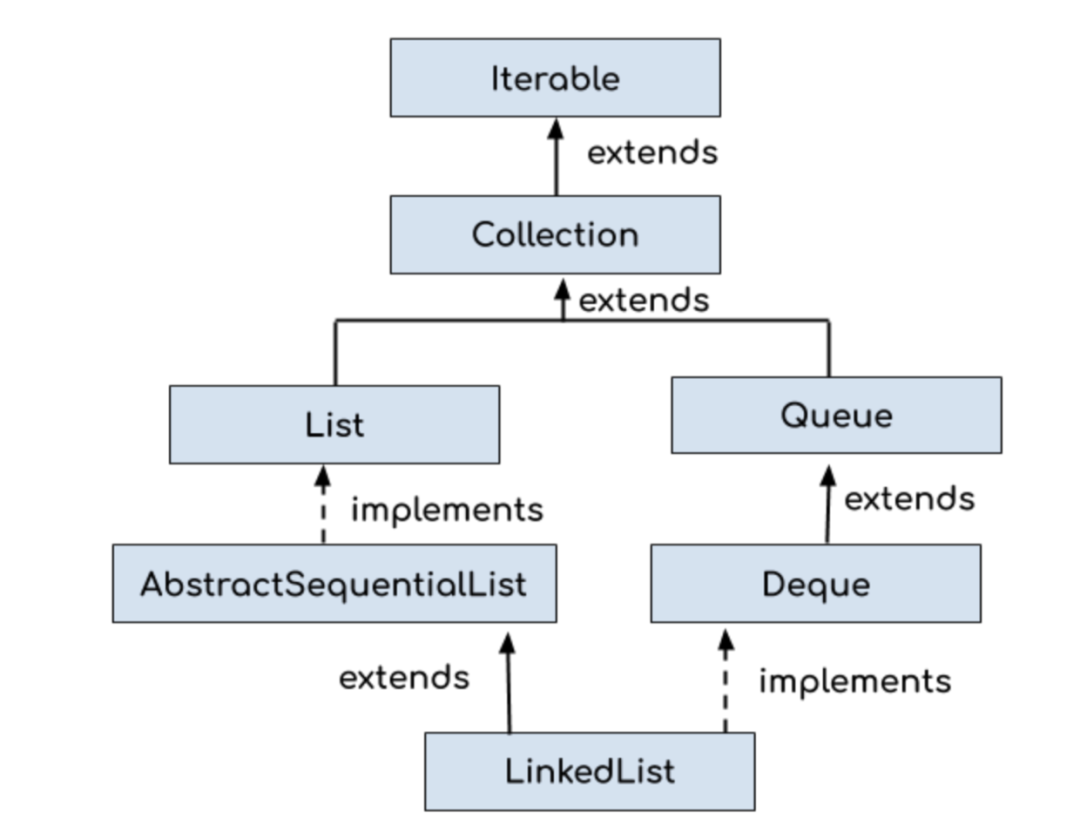public class LinkedList<E>    extends AbstractSequentialList<E>    implements List<E>, Deque<E>, Cloneable, java.io.Serializable{    transient int size = 0;    transient Node<E> first;    transient Node<E> last;}

LinkedList 内部定义了一个 Node 节点，它包含 3 个部分：元素内容 item，前引用 prev 和后引用 next。代码如下所示：

private static class Node<E> {    E item;    LinkedList.Node<E> next;    LinkedList.Node<E> prev;    Node(LinkedList.Node<E> prev, E element, LinkedList.Node<E> next) {        this.item = element;        this.next = next;        this.prev = prev;    }}

private void writeObject(java.io.ObjectOutputStream s)        throws java.io.IOException {    // Write out any hidden serialization magic    s.defaultWriteObject();    // Write out size    s.writeInt(size);    // Write out all elements in the proper order.    for (LinkedList.Node<E> x = first; x != null; x = x.next)        s.writeObject(x.item);}

private void readObject(java.io.ObjectInputStream s)        throws java.io.IOException, ClassNotFoundException {    // Read in any hidden serialization magic    s.defaultReadObject();    // Read in size    int size = s.readInt();    // Read in all elements in the proper order.    for (int i = 0; i < size; i++)        linkLast((E)s.readObject());}void linkLast(E e) {    final LinkedList.Node<E> l = last;    final LinkedList.Node<E> newNode = new LinkedList.Node<>(l, e, null);    last = newNode;    if (l == null)        first = newNode;    else        l.next = newNode;    size++;    modCount++;}

1）ArrayList

ArrayList 新增元素有两种情况，一种是直接将元素添加到数组末尾，一种是将元素插入到指定位置。

public boolean add(E e) {    modCount++;    add(e, elementData, size);    return true;}private void add(E e, Object[] elementData, int s) {    if (s == elementData.length)        elementData = grow();    elementData[s] = e;    size = s + 1;}

public void add(int index, E element) {    rangeCheckForAdd(index);    modCount++;    final int s;    Object[] elementData;    if ((s = size) == (elementData = this.elementData).length)        elementData = grow();    System.arraycopy(elementData, index,            elementData, index + 1,            s - index);    elementData[index] = element;    size = s + 1;}

public boolean add(E e) {    linkLast(e);    return true;}void linkLast(E e) {    final LinkedList.Node<E> l = last;    final LinkedList.Node<E> newNode = new LinkedList.Node<>(l, e, null);    last = newNode;    if (l == null)        first = newNode;    else        l.next = newNode;    size++;    modCount++;}

public void add(int index, E element) {    checkPositionIndex(index);    if (index == size)        linkLast(element);    else        linkBefore(element, node(index));}LinkedList.Node<E> node(int index) {    // assert isElementIndex(index);    if (index < (size >> 1)) {        LinkedList.Node<E> x = first;        for (int i = 0; i < index; i++)            x = x.next;        return x;    } else {        LinkedList.Node<E> x = last;        for (int i = size - 1; i > index; i--)            x = x.prev;        return x;    }}void linkBefore(E e, LinkedList.Node<E> succ) {    // assert succ != null;    final LinkedList.Node<E> pred = succ.prev;    final LinkedList.Node<E> newNode = new LinkedList.Node<>(pred, e, succ);    succ.prev = newNode;    if (pred == null)        first = newNode;    else        pred.next = newNode;    size++;    modCount++;}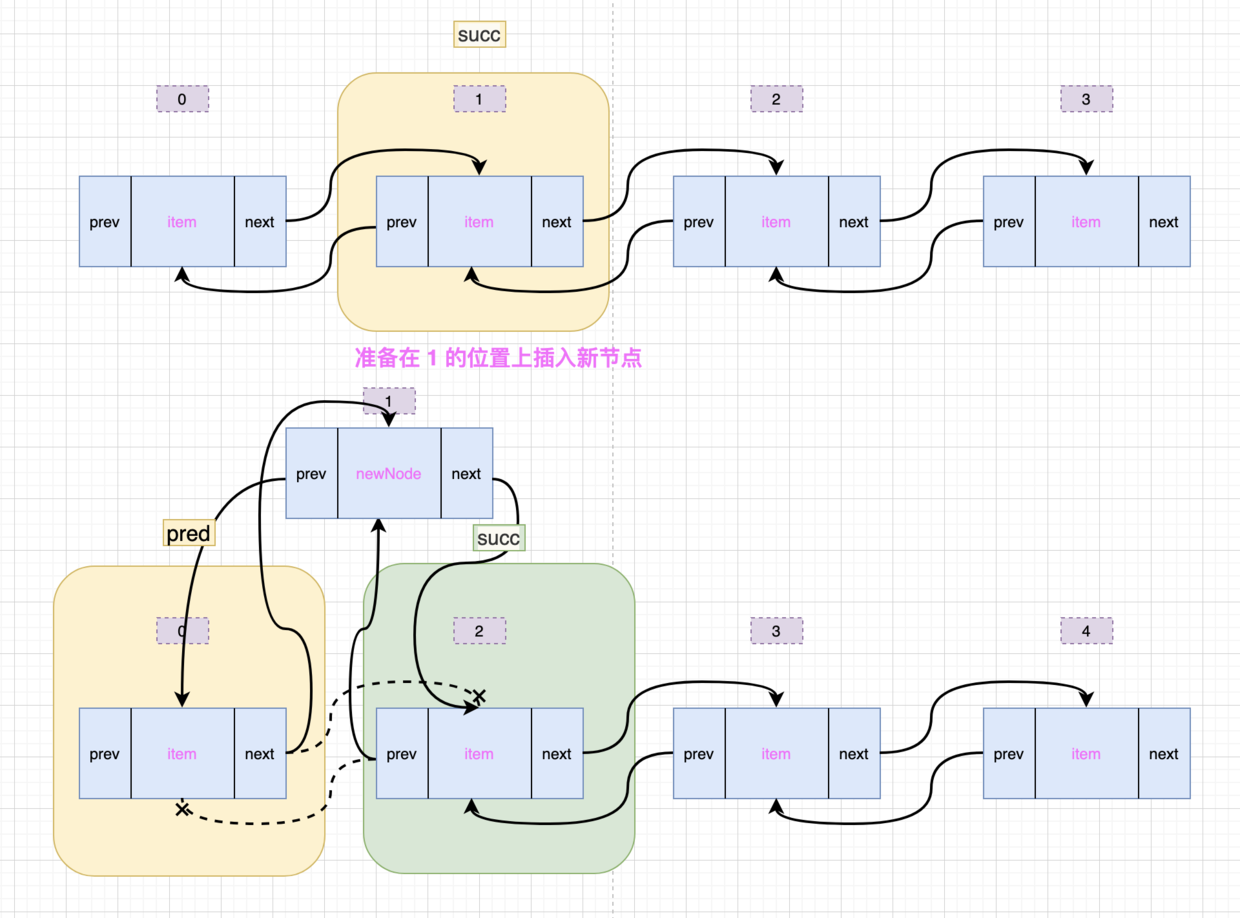public class ArrayListTest {    public static void addFromHeaderTest(int num) {        ArrayList<String> list = new ArrayList<String>(num);        int i = 0;        long timeStart = System.currentTimeMillis();        while (i < num) {            list.add(0, i + "沉默王二");            i++;        }        long timeEnd = System.currentTimeMillis();        System.out.println("ArrayList从集合头部位置新增元素花费的时间" + (timeEnd - timeStart));    }}/** * @author 微信搜「沉默王二」，回复关键字 PDF */public class LinkedListTest {    public static void addFromHeaderTest(int num) {        LinkedList<String> list = new LinkedList<String>();        int i = 0;        long timeStart = System.currentTimeMillis();        while (i < num) {            list.addFirst(i + "沉默王二");            i++;        }        long timeEnd = System.currentTimeMillis();        System.out.println("LinkedList从集合头部位置新增元素花费的时间" + (timeEnd - timeStart));    }}

num 为 10000，代码实测后的时间如下所示：

ArrayList从集合头部位置新增元素花费的时间595LinkedList从集合头部位置新增元素花费的时间15

public class ArrayListTest {    public static void addFromMidTest(int num) {        ArrayList<String> list = new ArrayList<String>(num);        int i = 0;        long timeStart = System.currentTimeMillis();        while (i < num) {            int temp = list.size();            list.add(temp / 2 + "沉默王二");            i++;        }        long timeEnd = System.currentTimeMillis();        System.out.println("ArrayList从集合中间位置新增元素花费的时间" + (timeEnd - timeStart));    }}public class LinkedListTest {    public static void addFromMidTest(int num) {        LinkedList<String> list = new LinkedList<String>();        int i = 0;        long timeStart = System.currentTimeMillis();        while (i < num) {            int temp = list.size();            list.add(temp / 2, i + "沉默王二");            i++;        }        long timeEnd = System.currentTimeMillis();        System.out.println("LinkedList从集合中间位置新增元素花费的时间" + (timeEnd - timeStart));    }}

num 为 10000，代码实测后的时间如下所示：

ArrayList从集合中间位置新增元素花费的时间1LinkedList从集合中间位置新增元素花费的时间101

public class ArrayListTest {    public static void addFromTailTest(int num) {        ArrayList<String> list = new ArrayList<String>(num);        int i = 0;        long timeStart = System.currentTimeMillis();        while (i < num) {            list.add(i + "沉默王二");            i++;        }        long timeEnd = System.currentTimeMillis();        System.out.println("ArrayList从集合尾部位置新增元素花费的时间" + (timeEnd - timeStart));    }}public class LinkedListTest {    public static void addFromTailTest(int num) {        LinkedList<String> list = new LinkedList<String>();        int i = 0;        long timeStart = System.currentTimeMillis();        while (i < num) {            list.add(i + "沉默王二");            i++;        }        long timeEnd = System.currentTimeMillis();        System.out.println("LinkedList从集合尾部位置新增元素花费的时间" + (timeEnd - timeStart));    }}

num 为 10000，代码实测后的时间如下所示：

ArrayList从集合尾部位置新增元素花费的时间69LinkedList从集合尾部位置新增元素花费的时间193

1）ArrayList

ArrayList 删除元素的时候，有两种方式，一种是直接删除元素（remove(Object)），需要直先遍历数组，找到元素对应的索引；一种是按照索引删除元素（remove(int)）。

public boolean remove(Object o) {    final Object[] es = elementData;    final int size = this.size;    int i = 0;    found: {        if (o == null) {            for (; i < size; i++)                if (es[i] == null)                    break found;        } else {            for (; i < size; i++)                if (o.equals(es[i]))                    break found;        }        return false;    }    fastRemove(es, i);    return true;}public E remove(int index) {    Objects.checkIndex(index, size);    final Object[] es = elementData;    @SuppressWarnings("unchecked") E oldValue = (E) es[index];    fastRemove(es, index);    return oldValue;}

private void fastRemove(Object[] es, int i) {    modCount++;    final int newSize;    if ((newSize = size - 1) > i)        System.arraycopy(es, i + 1, es, i, newSize - i);    es[size = newSize] = null;}

• remove(int)，删除指定位置上的元素

public E remove(int index) {    checkElementIndex(index);    return unlink(node(index));}

    E unlink(Node<E> x) {        // assert x != null;        final E element = x.item;        final Node<E> next = x.next;        final Node<E> prev = x.prev;        if (prev == null) {            first = next;        } else {            prev.next = next;            x.prev = null;        }        if (next == null) {            last = prev;        } else {            next.prev = prev;            x.next = null;        }        x.item = null;        size--;        modCount++;        return element;    }

• remove(Object)，直接删除元素

public boolean remove(Object o) {    if (o == null) {        for (LinkedList.Node<E> x = first; x != null; x = x.next) {            if (x.item == null) {                unlink(x);                return true;            }        }    } else {        for (LinkedList.Node<E> x = first; x != null; x = x.next) {            if (o.equals(x.item)) {                unlink(x);                return true;            }        }    }    return false;}

• removeFirst()，删除第一个节点

public E removeFirst() {    final LinkedList.Node<E> f = first;    if (f == null)        throw new NoSuchElementException();    return unlinkFirst(f);}private E unlinkFirst(LinkedList.Node<E> f) {    // assert f == first && f != null;    final E element = f.item;    final LinkedList.Node<E> next = f.next;    f.item = null;    f.next = null; // help GC    first = next;    if (next == null)        last = null;    else        next.prev = null;    size--;    modCount++;    return element;}

• removeLast()，删除最后一个节点

ArrayList从集合头部位置删除元素花费的时间380LinkedList从集合头部位置删除元素花费的时间4ArrayList从集合中间位置删除元素花费的时间381LinkedList从集合中间位置删除元素花费的时间5922ArrayList从集合尾部位置删除元素花费的时间8LinkedList从集合尾部位置删除元素花费的时间12

1）ArrayList

• get(int)，根据索引找元素

public E get(int index) {    Objects.checkIndex(index, size);    return elementData(index);}

• indexOf(Object)，根据元素找索引

public int indexOf(Object o) {    return indexOfRange(o, 0, size);}int indexOfRange(Object o, int start, int end) {    Object[] es = elementData;    if (o == null) {        for (int i = start; i < end; i++) {            if (es[i] == null) {                return i;            }        }    } else {        for (int i = start; i < end; i++) {            if (o.equals(es[i])) {                return i;            }        }    }    return -1;}

• get(int)，找指定位置上的元素

public E get(int index) {    checkElementIndex(index);    return node(index).item;}

• indexOf(Object)，找元素所在的位置

public int indexOf(Object o) {    int index = 0;    if (o == null) {        for (LinkedList.Node<E> x = first; x != null; x = x.next) {            if (x.item == null)                return index;            index++;        }    } else {        for (LinkedList.Node<E> x = first; x != null; x = x.next) {            if (o.equals(x.item))                return index;            index++;        }    }    return -1;}

LinkedList.Node<E> node(int index) {    // assert isElementIndex(index);    if (index < (size >> 1)) {        LinkedList.Node<E> x = first;        for (int i = 0; i < index; i++)            x = x.next;        return x;    } else {        LinkedList.Node<E> x = last;        for (int i = size - 1; i > index; i--)            x = x.prev;        return x;    }}

LinkedList<String> list = new LinkedList<String>();for (Iterator<String> it = list.iterator(); it.hasNext();) {    it.next();}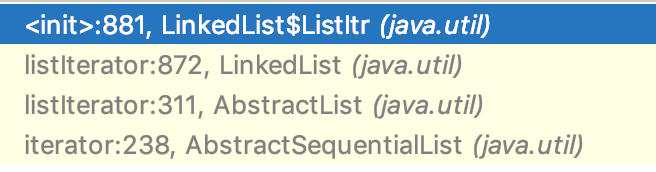public ListIterator<E> listIterator(int index) {    checkPositionIndex(index);    return new LinkedList.ListItr(index);}private class ListItr implements ListIterator<E> {    private LinkedList.Node<E> lastReturned;    private LinkedList.Node<E> next;    private int nextIndex;    private int expectedModCount = modCount;    ListItr(int index) {        // assert isPositionIndex(index);        next = (index == size) ? null : node(index);        nextIndex = index;    }    public boolean hasNext() {        return nextIndex < size;    }    public E next() {        checkForComodification();        if (!hasNext())            throw new NoSuchElementException();        lastReturned = next;        next = next.next;        nextIndex++;        return lastReturned.item;    }}

### 06、总结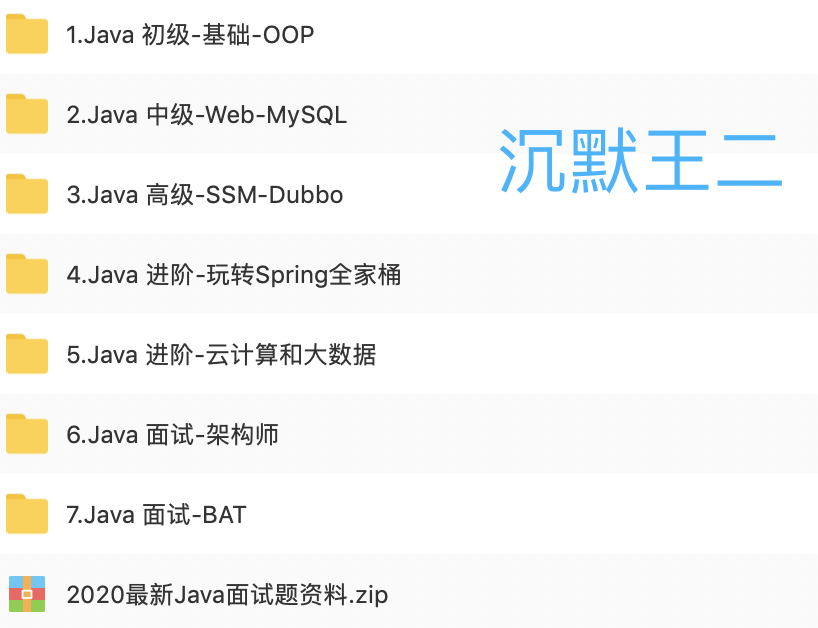### 沉默王二

《Web全栈开发进阶之路》作者 2018.07.31 加入

## 评论 (18 条评论)2020 年 09 月 29 日 17:43

2020 年 09 月 30 日 16:502020 年 09 月 29 日 15:24

2020 年 09 月 30 日 16:482020 年 09 月 29 日 09:24

2020 年 09 月 30 日 16:47infoQ也开始有这种培训软文了？
2020 年 09 月 28 日 11:57

2020 年 09 月 29 日 04:23

2020 年 09 月 30 日 15:22

2020 年 09 月 30 日 16:472020 年 09 月 28 日 10:49

2020 年 09 月 29 日 04:24

2020 年 09 月 29 日 08:56

2020 年 09 月 30 日 09:36